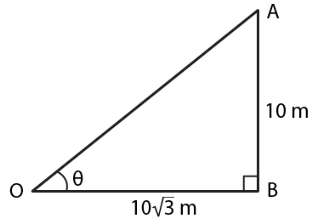Guru

# An electric pole is 10 metres high. If its shadow is 10√3 metres in length, find the elevation of the sun.

• 0

sir this is the question from the book -ML aggarwal( avichal publication) class 10th , chapter20 , heights and distances

we have been given that An electric pole is 10 metres high. If its shadow is 10√3 metres in length,  so we are asked to find the elevation of the sun.

question no 1 , heights and distances , ICSE board, M L Aggarwal

Share

1. Consider AB as the pole and OB as its shadow.

It is given that

AB = 10 m, OB = 10√3 m and θ is the angle of elevation of the sun.

We know that

tan θ = AB/ OB

Substituting the values

tan θ = 10/ 10√3 = 1/√3

So we get

tan 300 = 1/√3

θ = 300• 0